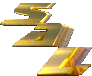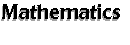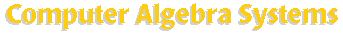[an error occurred while processing this directive] The major purpose of a Computer Algebra System (CAS) is to manipulate a formula symbolically using the computer. For example, expanding, factorizing, root finding, or simplifying an algebraic polynomial are some of the common uses of CAS. However, many systems listed here have gone far beyond that and also offer other functionalities like numerical calculation, graphics, and simulations. They are included in this section because their very original, and still their most distinguishable capability is performing computer algebra. Search SAL: Computer Algebra Systems   (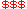Commercial,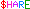Shareware,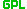GPL) Axiom-- a computer algebra system with powerful symbolic solver. bernina -- interactive program with interface to a computer algebra library. Computer Algebra Kit -- collection of small programs for computer algebra. CASA-- computer algebra software for constructive algebraic geometry in Maple. CoCoA -- a special-purpose system for doing Computations in Commutative Algebra. DrMath-- online symbolic math and computer algebra system. FELIX -- computations in and with algebraic structures and substructures. FORM -- symbolic manipulation for large mathematical problems. GAMS-- a high-level modeling system for mathematical programming problems. GAP -- computational discrete algebra emphasized on group theory. GiNaC-- open framework for symbolic computation within C++.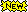GRG -- a computer algebra program for differential geometry, gravitation and field theory. GRTensorII -- computer algebra package for differential geometry. HartMath-- a computer algebra program written in Java. Kalamaris-- mathematical package similar to Mathematica. Interpreter for symbolic manipulation -- a simple computer algebra system in C++ or Java. JACAL-- symbolic simplification and manipulation of equations. lundin.SymbolicMath -- Java class library for symbolic differentiation and evaluation. Macaulay 2 -- algebraic geometry and commutative algebra. Macsyma-- a powerful and general purpose mathematical tools. MAGMA -- a system for algebra, number theory, geometry and combinatorics. Maple-- numerical computation, symbolic algebra, functions, graphics, programming. MAS -- experimental computer algebra system. Mathematica-- numerical computation, symbolic algebra, functions, graphics, programming. Mathomatic -- artificially intelligent algebraic manipualtor program. MAXIMA-- computer algebra system for symbolic and numerical computations. Symaxx -- a frontend for Maxima computer algebra system. Mock-Mma -- simple mock-up of Mathematica in Common Lisp. MuPAD -- symbolic and numeric computation, parallel programming and visualization. Punimax-- computer algebra system for symbolic and numerical computations. Reduce-- general algebraic computations. Redten -- symbolic algebra package for tensor objects. Ricci -- symbolic tensor computations for differential geometry. Risa/Asir -- experimental computer algebra system with programming libraries. SACLIB -- library of C programs for computer algebra. SAML-- a simple algebraic math library. SimLab -- CLISP code for computer algebra substrate and triangulations of 2D areas. Singular-- a computer algebra system for computing information about singularities. SISYPHOS -- computing in modular group algebras of p-groups. Software from Symbolic Computation Group -- for symbolic computation in physics. text_deriv -- a package designed to do simple symbolic derivatives. Yacas -- a small and highly flexible computer algebra language. Also Check Out: Algae-- a high-level interpreted language for numerical analysis. AUTOLEV-- a symbol manipulation program for analyzing the dynamics of mechanical systems. CAM C++ Class Libraries -- graphics, matrix/vector and symbolic function classes. FOAM-- computer algebra program for high energy physics. GNU Calc-- an advanced calculator that runs as part of the GNU Emacs environment.meditor-- a text editor with some symbolic computation capabilities. Pari/GP -- formal computations on recursive types at high speed (CA system in number theory). SHEEP -- a computer algebra system designed primarily for general relativity. SIMATH -- computer algebra system for number theory. Simple Lisp -- a simple lisp interpreter with symbolic math library for tensors. TMath -- a Tcl interface to Matlab and Mathematica. Other Useful Links Computer Algebra Information Network (CAIN) http://www.mupad.de/CAIN/index.html Symbolic Mathematical Computation Information Center http://symbolicnet.mcs.kent.edu/ Misc Symbolic Software Information from UC Berkeley ftp://math.berkeley.edu/pub/Symbolic_Soft/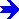Array-Oriented & Linear Algebra Systems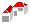SAL Home   |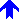Mathematics